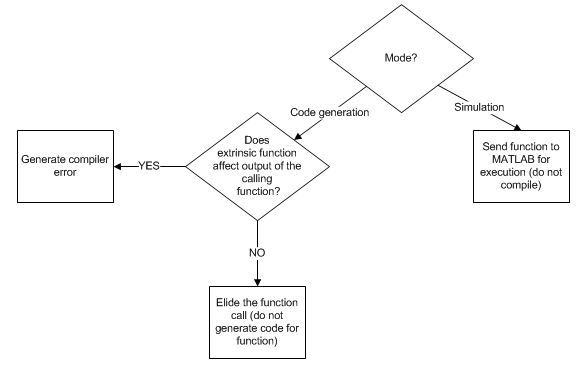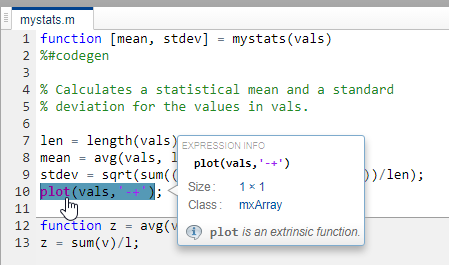Main Content

## Use MATLAB Engine to Execute a Function Call in Generated Code

When processing a call to a function `foo` in your MATLAB® code, the code generator finds the definition of `foo` and generates code for its body. In some cases, you might want to bypass code generation and instead use the MATLAB engine to execute the call. Use `coder.extrinsic('foo')` to declare that calls to `foo` do not generate code and instead use the MATLAB engine for execution. In this context, `foo` is referred to as an extrinsic function. This functionality is available only when the MATLAB engine is available during execution. Examples of such situations include execution of MEX functions, Simulink® simulations, or function calls at the time of code generation (also known as compile time).

If you generate standalone code for a function that calls `foo` and includes `coder.extrinsic('foo')`, the code generator attempts to determine whether `foo` affects the output. If `foo` does not affect the output, the code generator proceeds with code generation, but excludes `foo` from the generated code. Otherwise, the code generator produces a compilation error.

Including the `coder.extrinsic('foo')` directive inside a certain MATLAB function declares all calls to `foo` inside that MATLAB function as extrinsic. Alternatively, you might want to narrow the scope of extrinsic declaration to just one call to `foo`. See Calling MATLAB Functions Using feval.### When To Declare a Function as Extrinsic

These are some common situations in which you might consider declaring a MATLAB function as extrinsic:

• The function performs display or logging actions. Such functions are useful primarily during simulation and are not used in embedded systems.

• In your MEX execution or Simulink simulation, you want to use a MATLAB function that is not supported for code generation. This workflow does not apply to non-simulation targets.

• You instruct the code generator to constant fold a function call by using `coder.const`. In such situations, the function is called only during code generation when the MATLAB engine is available for executing the call.

### Using the `coder.extrinsic` Construct

To declare a function `foo` as extrinsic, include this statement in your MATLAB code.

`coder.extrinsic('foo')`

When declaring functions as extrinsic for code generation, adhere to these rules:

• Declare the function as extrinsic before you call it.

• Do not use the extrinsic declaration in conditional statements.

• Assign the return value of an extrinsic function to a known type. See Working with mxArrays.

For additional information and examples, see `coder.extrinsic`.

The code generator automatically treats many common MATLAB visualization functions, such as `plot`, `disp`, and `figure`, as extrinsic. You do not have to explicitly declare them as extrinsic functions by using `coder.extrinsic`. For example, you might want to call `plot` to visualize your results in the MATLAB environment. If you generate a MEX function from a function that calls `plot`, and then run the generated MEX function, the code generator dispatches calls to the `plot` function to the MATLAB engine. If you generate a library or executable, the generated code does not contain calls to the `plot` function.

If you generate MEX or standalone C/C++ code by using MATLAB Coder™, the code generation report highlights calls from your MATLAB code to extrinsic functions. By inspecting the report, you can determine which functions are supported only in the MATLAB environment.#### Scope of Extrinsic Function Declarations

The `coder.extrinsic` construct has function scope. For example, consider the following code:

```function y = foo %#codegen coder.extrinsic('rat','min'); [N D] = rat(pi); y = 0; y = min(N, D); ```

In this example, `rat` and `min` as treated as extrinsic every time they are called in the main function `foo`. There are two ways to narrow the scope of an extrinsic declaration inside the main function:

• Declare the MATLAB function extrinsic in a local function, as in this example:

```function y = foo %#codegen coder.extrinsic('rat'); [N D] = rat(pi); y = 0; y = mymin(N, D); function y = mymin(a,b) coder.extrinsic('min'); y = min(a,b); ```

Here, the function `rat` is extrinsic every time it is called inside the main function `foo`, but the function `min` is extrinsic only when called inside the local function `mymin`.

• Instead of using the `coder.extrinsic` construct, call the MATLAB function using `feval`. This approach is described in the next section.

#### Extrinsic Declaration for Nonstatic Methods

Suppose that you define a class `myClass` that has a nonstatic method `foo`, and then create an instance `obj` of this class. If you want to declare the method `obj.foo` as extrinsic in your MATLAB code that you intend for code generation, follow these rules:

• Write the call to `foo` as a function call. Do not write the call by using the dot notation.

• Declare `foo` to be extrinsic by using the syntax `coder.extrinsic('foo')`.

For example, define `myClass` as:

```classdef myClass properties prop = 1 end methods function y = foo(obj,x) y = obj.prop + x; end end end ```

Here is an example MATLAB function that declares `foo` as extrinsic.

```function y = myFunction(x) %#codegen coder.extrinsic('foo'); obj = myClass; y = foo(obj,x); end ```

Nonstatic methods are also known as ordinary methods. See Define Class Methods and Functions.

#### Additional Uses

Use the `coder.extrinsic` construct to:

• Call MATLAB functions that do not produce output during simulation, without generating unnecessary code.

• Make your code self-documenting and easier to debug. You can scan the source code for `coder.extrinsic` statements to isolate calls to MATLAB functions, which can potentially create and propagate `mxArrays`. See Working with mxArrays.

### Calling MATLAB Functions Using `feval`

To narrow the scope of extrinsic declaration to just one function call, use the function `feval`. `feval` is automatically interpreted as an extrinsic function during code generation. Therefore, you can use `feval` to conveniently call functions that you want to execute in the MATLAB environment, rather than compile to generated code.

Consider the following example:

```function y = foo coder.extrinsic('rat'); [N D] = rat(pi); y = 0; y = feval('min',N,D); ```

Because `feval` is extrinsic, the statement `feval('min',N,D)` is evaluated by MATLAB — not compiled — which has the same result as declaring the function `min` extrinsic for just this one call. By contrast, the function `rat` is extrinsic throughout the function `foo`.

The code generator does not support the use of `feval` to call local functions or functions that are located in a private folder.

### Working with mxArrays

The output of an extrinsic function is an `mxArray`, also known as a MATLAB array. The only valid operations for `mxArrays` are:

• Storing an `mxArray` in a variable.

• Passing an `mxArray` to a function.

• Returning an `mxArray` from a function back to MATLAB.

• Converting an `mxArray` to a known type at run time. To perform this action, assign the `mxArray` to a variable whose type is already defined by a prior assignment. See example below.

To use an `mxArray` returned by an extrinsic function in other operations (for example, returning it from a MATLAB Function block to Simulink execution), you must first convert it to a known type.

If the input arguments of a function are `mxArrays`, the code generator automatically treats the function as extrinsic.

#### Converting mxArrays to Known Types

To convert an `mxArray` to a known type, assign the `mxArray` to a variable whose type is defined. At run time, the `mxArray` is converted to the type of the variable that it is assigned to. However, if the data in the `mxArray` is not consistent with the type of the variable, you get a run-time error.

For example, consider this code:

```function y = foo %#codegen coder.extrinsic('rat'); [N D] = rat(pi); y = min(N,D); ```

Here, the top-level function `foo` calls the extrinsic MATLAB function `rat`, which returns two `mxArrays` representing the numerator `N` and denominator `D` of the rational fraction approximation of `pi`. You can pass these `mxArrays` to another MATLAB function — in this case, `min`. Because the inputs passed to `min` are `mxArrays`, the code generator automatically treats `min` as an extrinsic function. As a result, `min` returns an `mxArray`.

While generating a MEX function by using MATLAB Coder, you can directly assign this `mxArray` returned by `min` to the output `y` because the MEX function returns its output to MATLAB.

But if you put `foo` in a MATLAB Function block in a Simulink model and then update or run the model, you get this error:

```Function output 'y' cannot be an mxArray in this context. Consider preinitializing the output variable with a known type. ```

This error occurs because returning an `mxArray` back to Simulink is not supported. To fix this problem, define `y` to be the type and size of the value that you expect `min` to return — in this case, a scalar double — as follows:

```function y = foo %#codegen coder.extrinsic('rat'); [N D] = rat(pi); y = 0; % Define y as a scalar of type double y = min(N,D);```

### Restrictions on Using Extrinsic Functions

The full MATLAB run-time environment is not supported during code generation. Therefore, the following restrictions apply when calling MATLAB functions extrinsically:

• MATLAB functions that inspect the caller, or read or write to the caller workspace do not work during code generation. Such functions include:

• Functions in generated code can produce unpredictable results if your extrinsic function performs the following actions at run time:

• Change folders

• Change the MATLAB path

• Delete or add MATLAB files

• Change warning states

• Change MATLAB preferences

• Change Simulink parameters

• The code generator does not support the use of `coder.extrinsic` to call functions that are located in a private folder.

• The code generator does not support the use of `coder.extrinsic` to call local functions.

• You can call extrinsic functions with up to 64 inputs and 64 outputs.

## SupportGet trial now